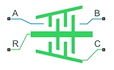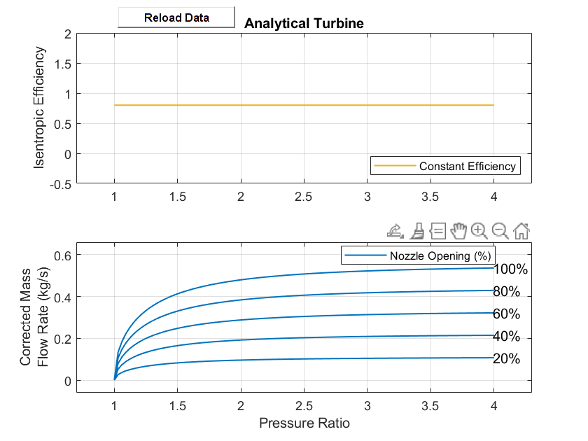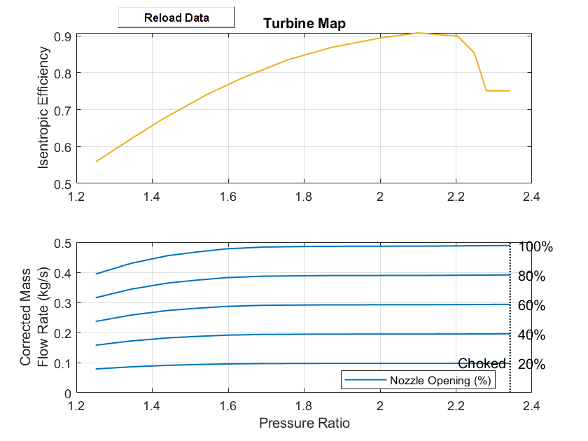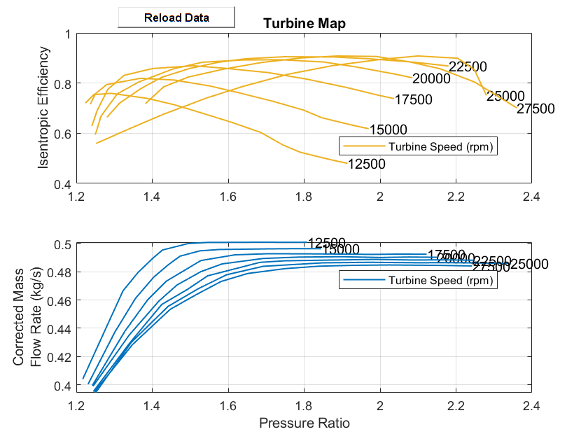# Turbine (2P)

Two-phase turbine in a thermodynamic cycle

• Library:
• Simscape / Fluids / Two-Phase Fluid / Fluid Machines

•## Description

The Turbine (2P) block models a turbine in a two-phase fluid network. You can parameterize the block as a tabulated turbine map that is either a 1-D function of pressure ratio or 2-D function of pressure ratio and corrected mass flow rate. Fluid flowing from port A to port B generates torque. Port R reports shaft torque and angular velocity relative to port C, which represents the turbine casing.

Port VN represents the vane opening fraction. This value linearly scales the corrected mass flow rate but does not influence the turbine efficiency. A value of `1` corresponds to the data in the turbine map.

The Turbine (2P) block assumes that superheated fluid enters the inlet. You can use the Report when fluid is not fully vapor parameter to choose what the block does when the fluid does not meet superheated conditions.

### Turbine Map

To visualize the block map, right-click the block and select Fluids > Plot Turbine Map.

Each time you modify the block settings, click on the figure window.

The turbine map relates turbine performance, as a function of pressure ratio, to the corrected mass flow rate and isentropic efficiency. The pressure ratio is the ratio between the turbine inlet pressure and outlet pressure.

The turbine map plots two separate graphs: corrected mass flow rate versus pressure ratio and isentropic efficiency versus pressure ratio. When you set the Turbine map parameterization to ``` Tabulated data - flow rate and efficiency vs. corrected speed and pressure ratio```, the plots also depend on the corrected rotor speed, N. The indexing variable β does not need to be the same between the mass flow rate and efficiency tables.

Corrected Mass Flow Rate

Due to the large changes in pressure and temperature inside a turbine, the turbine map plots performance in terms of the corrected mass flow rate. The block adjusts the corrected mass flow rate from the inlet mass flow rate by using a corrected pressure and temperature

`${\stackrel{˙}{m}}_{A}\sqrt{\frac{{T}_{A}}{{T}_{corr}}}={\stackrel{˙}{m}}_{corr}\frac{{p}_{A}}{{p}_{corr}},$`

where:

• $\stackrel{˙}{m}$A is the mass flow rate at port A.

• TA is the temperature at port A.

• Tcorr is the Reference temperature for corrected flow parameter. Refer to the data sheet for your turbine for this value.

• $\stackrel{˙}{m}$corr is the corrected mass flow rate.

When Turbine map parameterization is ```Analytical - nominal pressure ratio and corrected mass flow rate```, this is the value of the Nominal corrected mass flow rate parameter.

When Turbine map parameterization is ```Tabulated data - Flow rate and efficiency vs. pressure ratio```, this is the value of the Corrected mass flow rate vector, mdot(pr) parameter.

When Turbine map parameterization is ```Tabulated data - Flow rate and efficiency vs. corrected speed and pressure ratio```, this is the value of the Corrected mass flow rate table, mdot(N,beta) parameter.

• pA is the pressure at port A.

• pcorr is the Reference pressure for corrected flow parameter. Refer to the data sheet for your turbine for this value.

Corrected Speed

The block also adjusts the shaft speed, ω, according to the reference temperature, such that the corrected shaft speed is

`${\omega }_{corr}=\frac{\omega }{\sqrt{\frac{{T}_{A}}{{T}_{ref}}}}.$`

### Shaft Torque

The block calculates the shaft torque, τ, as

`$\tau =\frac{{\eta }_{m}{\stackrel{˙}{m}}_{A}\Delta {h}_{total}}{\omega },$`

where:

• Δhtotal is the total change in the fluid specific enthalpy.

• ηm is the value of the Mechanical efficiency parameter.

• ω is the relative shaft angular velocity, ωR - ωC.

Reversed flow, from port B to port A, is outside of the typical turbine operation mode and may not return accurate results. A threshold region when flow approaches zero ensures the block generates no torque when the flow rate is near zero or reversed.

### Analytical Parametrization

Use the analytical parametrization to create a turbine based on the expected nominal operating conditions. When Turbine map parameterization is ```Analytical – nominal pressure ratio and corrected mass flow rate```, the turbine isentropic efficiency is constant and the mass flow rate is a function of the pressure ratio.

This image shows the default turbine map when you use the analytical parameterization.### Parameterization by Pressure Ratio

When Turbine map parameterization is ```Tabulated data - Flow rate and efficiency vs. pressure ratio```, the turbine isentropic efficiency and mass flow rate are a function of the pressure ratio and do not account for any changes in the turbine rotational speed.

The last element of the Corrected mass flow rate vector, mdot(pr) and the Pressure ratio vector, pr parameters represents the corrected mass flow rate and pressure ratio at choked flow conditions.

This image shows the default turbine map when you use the 1-D parameterization.### Parameterization by Pressure Ratio and Corrected Mass Flow Rate

When Turbine map parameterization is set to ```Tabulated data - Flow rate and efficiency vs. corrected speed and pressure ratio```, the turbine isentropic efficiency, pressure ratio, and corrected mass flow rate are a function of the corrected speed, N, and the map index, β. The block uses linear interpolation between data points for the efficiency, pressure ratio, and corrected mass flow rate parameters.

The last column of the Corrected mass flow rate table, mdot(N,beta) and the Pressure ratio table, pr(N,beta) represent the corrected mass flow rate and pressure ratio at choked flow conditions.

This image shows the default turbine map when you use the 2-D parameterization.### Continuity Equations

Mass is preserved over the block:

`${\stackrel{˙}{m}}_{A}+{\stackrel{˙}{m}}_{B}=0,$`

where $\stackrel{˙}{m}$B is the mass flow rate at port B.

The block calculates the energy balance as

`${\Phi }_{A}+{\Phi }_{B}-{P}_{fluid}=0,$`

where:

• ΦA is the energy flow rate at port A.

• ΦB is the energy flow rate at port B.

• Pfluid is the work done by the fluid, which the block determines from the change in total fluid specific enthalpy, ${P}_{fluid}={\stackrel{˙}{m}}_{A}\Delta {h}_{total}.$

### Assumptions and Limitations

• The shaft does not rotate under reversed flow conditions. Results during reversed flows may not be accurate.

## Ports

### Input

expand all

Vane opening fraction, specified as a unitless physical signal. The corrected mass flow rate scales linearly with the value of VN. A fraction of `1` corresponds to the tabulated data. The vane opening fraction does not influence the isentropic efficiency.

### Conserving

expand all

Two-phase fluid conserving port associated with the fluid entry port.

Two-phase fluid conserving port associated with the fluid exit port.

Port associated with the turbine casing.

Port associated with the turbine shaft torque and angular velocity.

## Parameters

expand all

### Parameterization

Performance parameterization specification:

• ```Analytical - Nominal pressure ratio and corrected mass flow rate```: Parameterize turbine isentropic efficiency and corrected mass flow rate analytically.

• ```Tabulated data - Flow rate and efficiency vs. pressure ratio```: Parameterize turbine isentropic efficiency and corrected mass flow rate by pressure ratio.

• ```Tabulated data - Flow rate and efficiency vs. corrected speed and pressure ratio```: Parameterize turbine isentropic efficiency and corrected mass flow rate by corrected speed and pressure ratio.

Minimum vane opening fraction. This value is the lower boundary to the signal at VN. The block multiplies the value of this parameter by the mass flow rate to obtain the minimum corrected mass flow rate through the turbine.

Maximum vane opening. This value is the upper boundary to the signal at VN. The block multiplies the value of this parameter by the mass flow rate to obtain the maximum corrected mass flow rate through the turbine.

Whether the block does nothing, generates a warning, or generates an error when the block detects fluid that is not fully vapor.

Vector of constant shaft speeds for tabular parameterization of corrected mass flow rate and isentropic efficiency.

#### Dependencies

To enable this parameter, set Turbine map parameterization to ```Tabulated data - Flow rate and efficiency vs. corrected speed and pressure ratio```.

### Flow Rate Data

Pressure ratio value under nominal operating conditions for the analytical parameterization.

#### Dependencies

To enable this parameter, set Turbine map parameterization to ```Analytical - Nominal pressure ratio and corrected mass flow rate```.

Corrected mass flow rate value under nominal operating conditions for the analytical parameterization.

#### Dependencies

To enable this parameter, set Turbine map parameterization to ```Analytical - Nominal pressure ratio and corrected mass flow rate```.

Vector of pressure ratio values for the tabulated parameterization of corrected mass flow rate. This vector must have the same number of elements as the Corrected mass flow rate vector, mdot(pr) parameter and all elements must be greater than 1. The last element must be the pressure ratio at choked conditions.

#### Dependencies

To enable this parameter, set Turbine map parameterization to ```Tabulated data - Flow rate and efficiency vs. pressure ratio```.

Vector of corrected mass flow rate values for the tabulated parameterization of corrected mass flow rate. This vector must have the same number of elements as the Pressure ratio vector, pr parameter and all elements must be greater than 0. The last element must be the corrected mass flow rate at choked conditions.

#### Dependencies

To enable this parameter, set Turbine map parameterization to ```Tabulated data - Flow rate and efficiency vs. pressure ratio```.

M-by-N matrix of turbine outlet-to-inlet pressure ratios at the specified corrected shaft speed and β value. The block employs linear interpolation between the table elements. M and N are the sizes of the corresponding vectors:

• M is the number of vector elements in the Corrected speed index vector, N parameter.

• N is the number of β indices in the table.

The default table values are:

```[1.217, 1.285, 1.323, 1.363, 1.402, 1.427, 1.495, 1.545, 1.6, 1.687, 1.747, 1.8074; 1.231, 1.302, 1.356, 1.404, 1.443, 1.473, 1.525, 1.577, 1.635, 1.709, 1.782, 1.845; 1.242, 1.328, 1.388, 1.437, 1.528, 1.617, 1.704, 1.811, 1.915, 1.979, 2.053, 2.1226; 1.244, 1.339, 1.417, 1.465, 1.528, 1.584, 1.692, 1.787, 1.899, 2, 2.075, 2.1492; 1.245, 1.34, 1.425, 1.492, 1.546, 1.684, 1.748, 1.882, 1.987, 2.084, 2.167, 2.2458; 1.251, 1.345, 1.442, 1.521, 1.596, 1.692, 1.849, 1.972, 2.1, 2.173, 2.259, 2.3424; 1.248, 1.348, 1.447, 1.516, 1.581, 1.651, 1.749, 1.839, 1.93, 2.024, 2.154, 2.242]```

#### Dependencies

To enable this parameter, set Turbine map parameterization to ```Tabulated data - Flow rate and efficiency vs. corrected speed and pressure ratio```.

M-by-N matrix of corrected mass flow rates at the specified corrected shaft speed and β value. The block employs linear interpolation between the table elements. M and N are the sizes of the corresponding vectors:

• M is the number of vector elements in the Constant speed index vector, N parameter.

• N is the number of β indices in the table.

The default table values are:

```0.01.*[40.403, 44.417, 46.653, 47.966, 48.97, 49.544, 50.001, 50.081, 50.086, 50.095, 50.09, 50.0922; 40.044, 43.754, 46.125, 47.626, 48.513, 49.105, 49.481, 49.58, 49.597, 49.624, 49.638, 49.633; 39.941, 43.795, 46.017, 47.335, 48.795, 49.199, 49.279, 49.27, 49.248, 49.23, 49.255, 49.2428; 39.896, 43.512, 45.914, 47.061, 48.011, 48.558, 48.943, 49.042, 49.069, 49.01, 49.046, 49.0266; 39.524, 42.934, 45.686, 46.743, 47.715, 48.67, 48.777, 48.836, 48.876, 48.836, 48.845, 48.8184; 39.439, 43.01, 45.556, 46.801, 47.792, 48.388, 48.634, 48.67, 48.639, 48.647, 48.625, 48.5912; 39.502, 42.849, 45.336, 46.385, 47.312, 47.877, 48.222, 48.397, 48.459, 48.482, 48.455, 48.414]``` kg/s

#### Dependencies

To enable this parameter, set Turbine map parameterization to ```Tabulated data - Flow rate and efficiency vs. corrected speed and pressure ratio```.

### Efficiency Data

Isentropic efficiency under nominal operating conditions for the analytical parametrization.

#### Dependencies

To enable this parameter, set Turbine map parameterization to ```Analytical - Nominal pressure ratio and corrected mass flow rate```.

Vector of pressure ratio values for the tabulated parameterization of isentropic efficiency. This vector must have the same number of elements as the Isentropic efficiency vector, eta(pr)) parameter and all elements must be greater than 1.

#### Dependencies

To enable this parameter, set Turbine map parameterization to ```Tabulated data - Flow rate and efficiency vs. pressure ratio```.

Vector of isentropic efficiency values for the tabulated parameterization of isentropic efficiency. This vector must have the same number of elements as the Pressure ratio vector, pr parameter and all elements must be greater than 0 and less than or equal to 1.

#### Dependencies

To enable this parameter, set Turbine map parameterization to ```Tabulated data - Flow rate and efficiency vs. pressure ratio```.

M-by-N matrix of turbine outlet-to-inlet pressure ratios at the specified corrected shaft speed and β value. The block employs linear interpolation between the table elements. M and N are the sizes of the corresponding vectors:

• M is the number of vector elements in the Constant speed index vector, N parameter.

• N is the number of β indices in the table.

The default table values are:

```[1.224, 1.246, 1.292, 1.387, 1.457, 1.534, 1.61, 1.686, 1.743, 1.79, 1.871, 1.914; 1.237, 1.25, 1.28, 1.369, 1.459, 1.553, 1.629, 1.716, 1.799, 1.846, 1.927, 1.97; 1.241, 1.255, 1.283, 1.326, 1.401, 1.509, 1.591, 1.704, 1.806, 1.913, 1.994, 2.037; 1.25, 1.265, 1.308, 1.364, 1.456, 1.532, 1.637, 1.739, 1.851, 1.934, 2.042, 2.085; 1.281, 1.314, 1.371, 1.425, 1.498, 1.574, 1.647, 1.749, 1.878, 1.979, 2.091, 2.18; 1.252, 1.328, 1.425, 1.544, 1.642, 1.757, 1.873, 2.005, 2.1, 2.204, 2.248, 2.28; 1.382, 1.43, 1.495, 1.586, 1.688, 1.791, 1.887, 1.99, 2.079, 2.158, 2.249, 2.217]```

#### Dependencies

To enable this parameter, set Turbine map parameterization to ```Tabulated data - Flow rate and efficiency vs. corrected speed and pressure ratio```.

M-by-N matrix of turbine isentropic efficiencies at the specified constant shaft speed and β value. The block employs linear interpolation between the table elements. M and N are the sizes of the corresponding vectors:

• M is the number of vector elements in the Constant speed index vector, N parameter.

• N is the number of β indices in the table.

The default table values are:

```[.72, .754, .759, .739, .714, .679, .643, .603, .554, .524, .494, .48; .716, .757, .794, .819, .812, .788, .761, .728, .692, .662, .632, .618; .629, .691, .775, .831, .858, .869, .859, .842, .816, .782, .752, .738; .594, .668, .757, .804, .857, .883, .892, .893, .884, .865, .835, .821; .664, .72, .779, .823, .858, .883, .897, .905, .904, .896, .883, .868; .559, .61, .673, .741, .788, .835, .869, .895, .908, .899, .854, .751; .718, .785, .824, .855, .882, .9, .908, .906, .884, .857, .804, .701]```

#### Dependencies

To enable this parameter, set Turbine map parameterization to ```Tabulated data - Flow rate and efficiency vs. corrected speed and pressure ratio```.

### Reference Data

Pressure at which the turbine data consolidates to a corrected trend line.

Temperature at which the turbine data consolidates to a corrected trend line.

Efficiency of the conversion from reduction in specific enthalpy to shaft torque.

Turbine inlet cross-sectional area.

Turbine outlet cross-sectional area.

 Kurzke, Joachim. "How to Get Component Maps for Aircraft Gas Turbine Performance Calculations." Volume 5: Manufacturing Materials and Metallurgy; Ceramics; Structures and Dynamics; Controls, Diagnostics and Instrumentation; Education; General, American Society of Mechanical Engineers, 1996, p. V005T16A001.

 Plencner, Robert M. “Plotting component maps in the Navy/NASA Engine Program (NNEP): A method and its usage.” NASA Technical Memorandum, 1989.